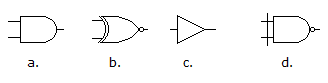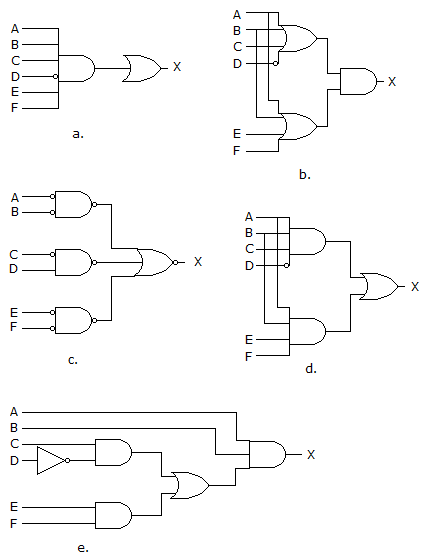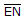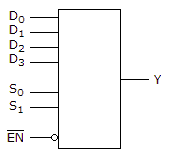# Digital Electronics - Combinational Logic Circuits

Exercise : Combinational Logic Circuits - General Questions
1.
How many 3-line-to-8-line decoders are required for a 1-of-32 decoder?
1
2
4
8
Explanation:
No answer description is available. Let's discuss.

2.

Which of the figures shown below represents the exclusive-NOR gate?a
b
c
d
Explanation:
No answer description is available. Let's discuss.

3.

Which of the circuits in figure (a to d) is the sum-of-products implementation of figure (e)?a
b
c
d
Explanation:
No answer description is available. Let's discuss.

4.

For the device shown here, let all D inputs be LOW, both S inputs be HIGH, and theinput be LOW. What is the status of the Y output?LOW
HIGH
Don't Care
Cannot be determined
Explanation:
No answer description is available. Let's discuss.

5.

For the device shown here, let all D inputs be LOW, both S inputs be HIGH, and theinput be HIGH. What is the status of the Y output?LOW
HIGH
Don't Care
Cannot be determined